This video was brought to you by GeneralPAC.com, making power systems Intuitive, Open and Free for Everyone, Everywhere. Consider subscribing and supporting through patreon.com/GeneralPAC. This is a mechanism for you to support us financially so we can continue making high quality power system video tutorials. Our corporate sponsor for this topic is AllumiaX.com from Seattle, Washington. Contact them for industrial and commercial power system studies.

In part 1b of the series, we shall continue from our previous part, and talk about excitation current and the excitation curve in much more detail.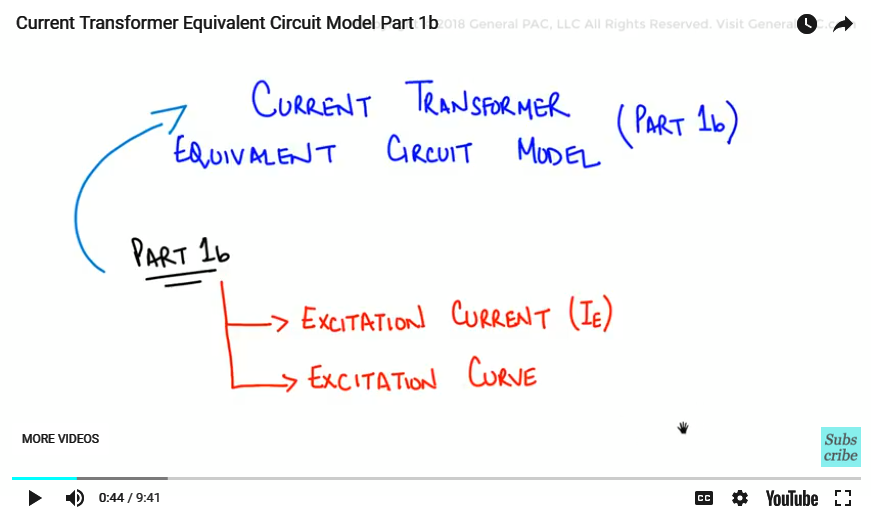Text BoxShapeNow, let’s begin by considering the parameter that we have discussed before in much greater detail, which is the Excitation current, “IE”. Now, this parameter as described before is responsible for the excitation of the CT core. It actually makes the CT work in real life.

But it is also the current that lost through the magnetizing branch and corresponds to CT error. So, the more the excitation current (IE) the more the errors or the more saturation that the CT will observe. Now the value of this excitation current should be kept as minimum as possible as explain in the previous tutorial.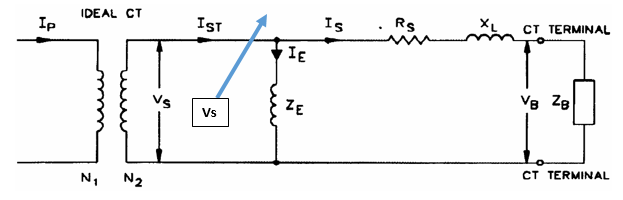Now the CT operation can be easily explained by using the Excitation curve. Now, this curves shows the relation ; It shows the relationship between the voltage across the burden (VB) and the excitation current (IE). Vs is the voltage across the excitation branch as pointed out by this Diagram and also sometimes described as VE as an echo.

so, we just need to consider these values in a little bit more detail.

Now, keep in mind that the voltage across the burden is (Vb) and there is the difference between the voltage across the excitation branch, which we are calling (Vs) and the voltage across the burden and the difference comes from the fact that we have this Rs and Xl, the secondary winding resistance plus the leakage reactance that’s how we are modeling it. Now, the difference here, how we calculated is if we wanted to calculate the voltage across the burden. Well, that simply equals the voltage across or the secondary winding voltage Vs.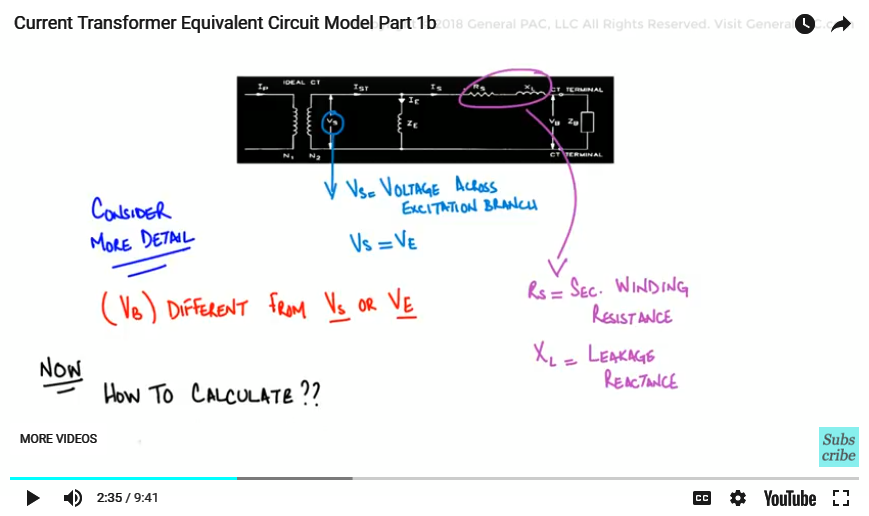Another way of saying is that it is the voltage across the excitation impedance minus the voltage dropped across Rs + (Xl).

Right, which is the current going through (Rs and Xl) times the impedance of Rs + Xl. So that is represented by the equation that you see.

VB= Vs – Is*(Rs + Xl)

Let’s now consider an example of a CT excitation curve.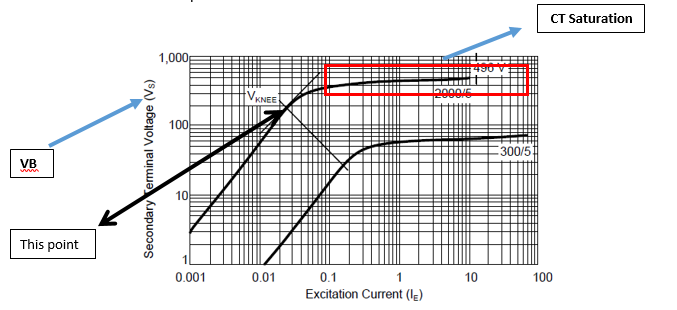The excitation curve that we see here is the C400, 2000:5 multi-ratios current transformer. We see that; it is multi-ratio because we see two curves and one curve is represents 2000:5 and other curve represents 300:5.

Okay, now how would we know what the CT rating is? Well, we can approximate the CT rating pretty easily. So what we do, is we look at and we will go over this in much more detail, but what we do, we look at the excitation current which is the X-axis and we look at the secondary terminal voltage (Vs) which is at the Y-axis.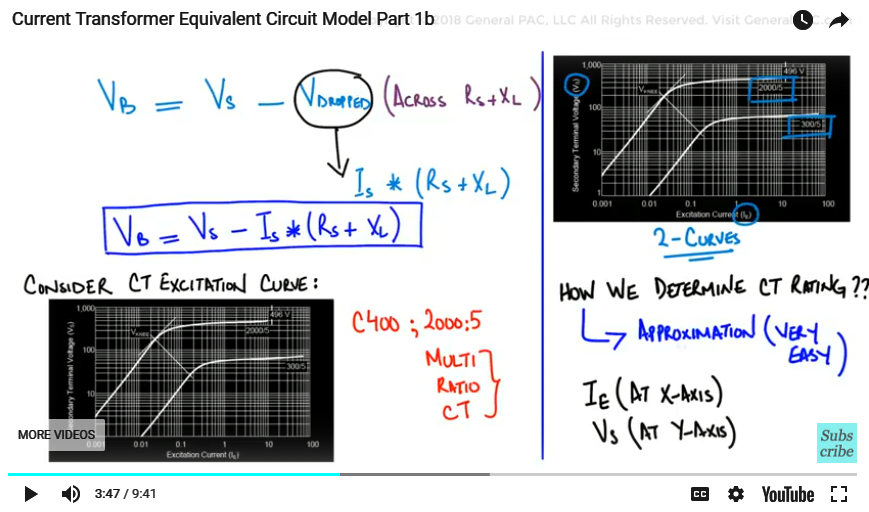Again the secondary terminal voltage is the same thing as the voltage across the burden which is the really important parameter. Okay, so we need to find a way to use those two axis to determine what CT rating is? Meaning if it’s C400, C800, C100…..how do we know?

Okay, so the rule of thumb is this, we look at the excitation current and we look at that mark where it has 10. At that particular point where does the excitation curve goanna meet, so we look at the 10 and we go all over up and we find that its approximately 496 volts.

So 496, is more than 400, so what we can say that it is definitely not the C800, not a C600 and it probably won’t qualify for a C500 either. But we can say that, yeah it is C400 with a quite bit of margin. So hat is the quite an approximate way to determine what type of CT ratings it would be?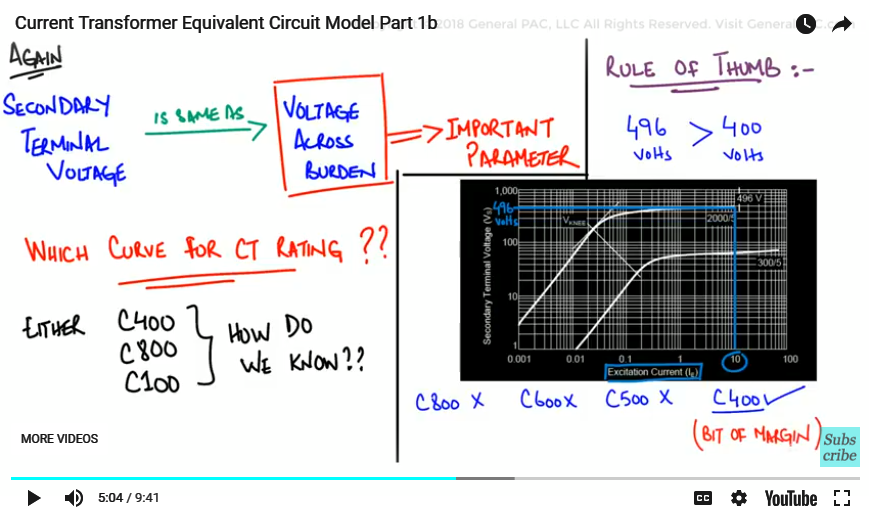Okay, so we can go through this in much more detail, if there is an interest of that. Now the interesting thing here Is that the second curve that we see that is the ratio at 300:5 is a multi-ratio current transformer. That means, that if we are looking at a ratio that is not the full ratio meaning it is a tap ratio then the rating of a current transformer changes and so in this example at 10 Amps exciting current, it’s definitely less than 100 volts

As, we change the ratio, we also change the rating quite considerably. So keep that in mind!

Now, the excitation impedance can be obtained by dividing each value of voltage that we see on the graph with the corresponding excitation current, and this results in the table that we see below.

Now, in this table, what’s interesting here is that the excitation impedance, we see that it’s a non-linear value. It increases from 3000 ohms at 0.001 Amps excitation current to a maximum of 8065 ohms at 0.025 amps exciting current. Now this is the point of maximum permeability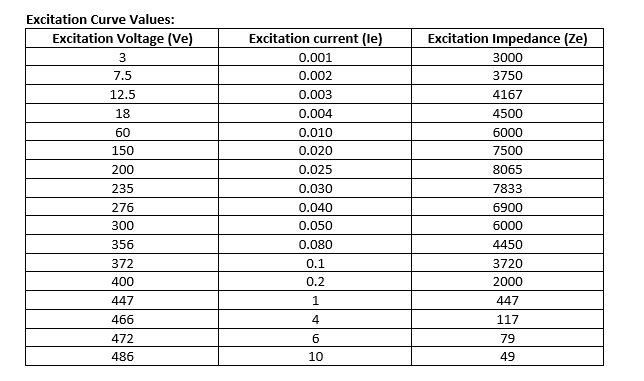And it is the same point that is shown on the graph by drawing tangent to the curve. This is the point that is termed as the “Knee Point” of the curve. Now the impedance value that starts to saturate from that point towards onward, meaning that once the impedance reaches that 49 ohms at 10 Amps exciting current.

Anything beyond that, or anything beyond the knee-point of the curve, it becomes to give more errors. Now to summarize this discussion and I know I will talking about this in much more detail later.

We can say, that the secondary voltage of the CT increases, then the exciting current increases as well. But only to the point of the Knee curve and beyond that exciting current stabilizes to the constant value.

We can observe a linear relation, right the linear relationship or proportional relationship between the terminal voltage (VB) or the voltage across the burden and the excitation current (IE) up to the Knee-point of the curve. The point is called the “knee-point voltage”.

This is a very very important parameter but this is the exact same point where the CT starts to exhibit nonlinear behavior, where a 10 percent increase in the secondary voltage causes an increase of the secondary current by 50 percent..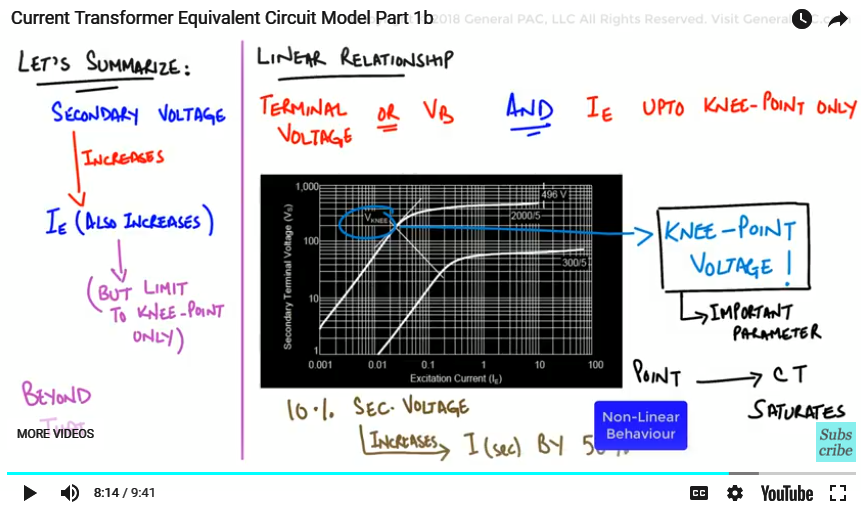So, therefore, if we go above the knee-point of the curve, the exciting current will be abnormally high and it’s required to keep excitation current as minimum as possible, in order to avoid saturation.

So, anything above the knee-point of the curve, will goanna exhibit saturation. Okay, because the exciting current becomes increases by a log scale. Right, so increase in the small change in voltage, will have a huge change in the current, the way that the characteristics laid out.

Now this example is derived from the S. E. L’s article called “Beyond the Knee-point: A practical guide to CT Saturation”. So, rest assured, these are good and accurate examples. If mistakes are found, they will be described at generalpac.com

Now, in the next part, we will be discussing the CT Burden and the CT class ratings in much greater detail.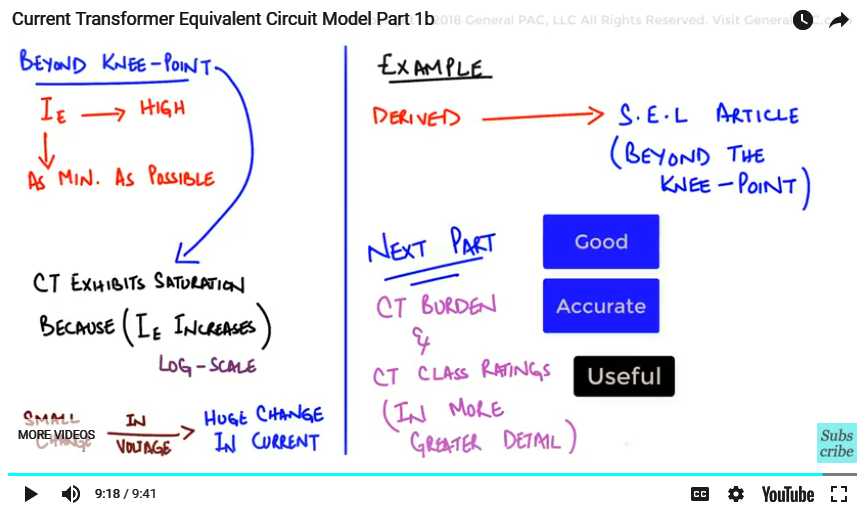Now, if you find this topic useful and enjoyable. Regardless if you are student and professional. Please do consider subscribing to GeneralPac.com and consider becoming our Patron on Patreon.com/generalpac. That way, we can continue to create these really high quality technical power systems video tutorials free for everyone and everywhere.

#### Greetings from the GeneralPAC Team!

We make high-quality Power Systems Video Tutorials on complex topics that are free and open to everyone!  Thank you so much for supporting us through Patreon so we can continue doing good and valuable work.

What is Patreon and why do we use it?

Patreon is a fantastic portal that allows our fans and community to make monthly contribution (like Netflix subscription) so we can continue creating high-quality power systems video tutorials. In return, you get access to incredible perks like voting on future topics, getting your questions answered, access to VIP Q/A webinars with the creators of GeneralPAC, and much more! We THANK YOU for supporting us

Why do we need your support?

An incredible amount of time and effort is needed to develop high-quality video tutorials. Each video (Part 1 for example) takes approximately 10 hours to complete which includes learning the concept ourselves, brainstorming creative ways to teach and explain the concepts, writing the script, audio recording, video recording, and editing. It's no wonder why Hundreds-of-Thousands of people have watched, liked, subscribed, and left positive comments on Youtube channel. Your support truly makes all the difference.